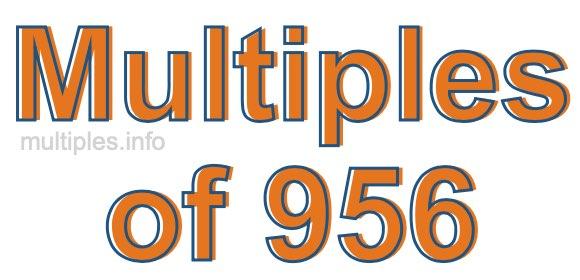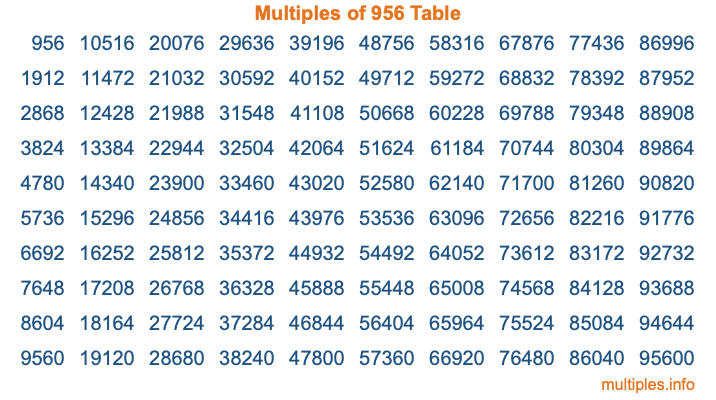Multiples of 956Welcome to the Multiples of 956 page. Here we will first teach you everything you will ever need to know about the multiples of 956, and then give you a study guide summary of everything we taught you to make sure you remember it all. Use this page to look up facts and learn information about the multiples of 956. This page will make you a multiples of nine hundred fifty-six expert!

Definition of Multiples of 956
Multiples of 956 are all the numbers that when divided by 956 equal an integer. Each of the multiples of 956 are called a multiple. A multiple of 956 is created by multiplying 956 by an integer.

Therefore, to create a list of multiples of 956, you start with 1 multiplied by 956, then 2 multiplied by 956, then 3 multiplied by 956, and so on for as long as you want. Thus, the list of the first five multiples of 956 is 956, 1912, 2868, 3824, and 4780. To see a larger list of multiples of 956, see the printable image of Multiples of 956 further down on this page. We also have a category where you can choose any nth multiple of 956.

Multiples of 956 Checker
The Multiples of 956 Checker below checks to see if any number of your choice is a multiple of 956. In other words, it checks to see if there is any number (integer) that when multiplied by 956 will equal your number. To do that, we divide your number by 956. If the the quotient is an integer, then your number is a multiple of 956.

Is  a multiple of 956?

Least Common Multiple of 956 and ...
A Least Common Multiple (LCM) is the lowest multiple that two or more numbers have in common. This is also called the smallest common multiple or lowest common multiple and is useful to know when you are adding our subtracting fractions. Enter one or more numbers below (956 is already entered) to find the LCM.

Check out our LCM Calculator if you need more details about the Least Common Multiple or if you need the LCM for different numbers for adding and subtraction fractions.

nth Multiple of 956
As we stated above, 956 is the first multiple of 956, 1912 is the second multiple of 956, 2868 is the third multiple of 956, and so on. Enter a number below to find the nth multiple of 956.

th multiple of 956

Multiples of 956 vs Factors of 956
956 is a multiple of 956 and a factor of 956, but that is where the similarities end. All postive multiples of 956 are 956 or greater than 956. All positive factors of 956 are 956 or less than 956.

Below is the beginning list of multiples of 956 and the factors of 956 so you can compare:

Multiples of 956: 956, 1912, 2868, 3824, 4780, etc.

Factors of 956: 1, 2, 4, 239, 478, 956

As you can see, the multiples of 956 are all the numbers that you can divide by 956 to get a whole number. The factors of 956, on the other hand, are all the whole numbers that you can multiply by another whole number to get 956.

It's also interesting to note that if a number (x) is a factor of 956, then 956 will also be a multiple of that number (x).

Multiples of 956 vs Divisors of 956
The divisors of 956 are all the integers that 956 can be divided by evenly. Below is a list of the divisors of 956.

Divisors of 956: 1, 2, 4, 239, 478, 956

The interesting thing to note here is that if you take any multiple of 956 and divide it by a divisor of 956, you will see that the quotient is an integer.

Multiples of 956 Table
Below is an image of the first 100 multiples of 956 in a table. The table is in chronological order, column by column. The first column has the first ten multiples of 956, the second column has the next ten multiples of 956, and so on.The Multiples of 956 Table is also referred to as the 956 Times Table or Times Table of 956. You are welcome to print out our table for your studies.

Negative Multiples of 956
Although not often discussed or needed in math, it is worth mentioning that you can make a list of negative multiples of 956 by multiplying 956 by -1, then by -2, then by -3, and so on, to get the following list of negative multiples of 956:

-956, -1912, -2868, -3824, -4780, etc.

Multiples of 956 Summary
Below is a summary of important Multiples of 956 facts that we have discussed on this page. To retain the knowledge on this page, we recommend that you read through the summary and explain to yourself or a study partner why they hold true.

There are an infinite number of multiples of 956.

A multiple of 956 divided by 956 will equal a whole number.

956 divided by a factor of 956 equals a divisor of 956.

The nth multiple of 956 is n times 956.

The largest factor of 956 is equal to the first positive multiple of 956.

956 is a multiple of every factor of 956.

956 is a multiple of 956.

A multiple of 956 divided by a divisor of 956 equals an integer.

956 divided by a divisor of 956 equals a factor of 956.

Any integer times 956 will equal a multiple of 956.

Multiples of a Number
Here you can get the multiples of another number, all with the same attention to detail as we did for multiples of 956 on this page.

Multiples of
Multiples of 957
Did you find our page about multiples of nine hundred fifty-six educational? Do you want more knowledge? Check out the multiples of the next number on our list!